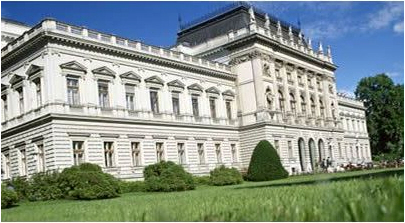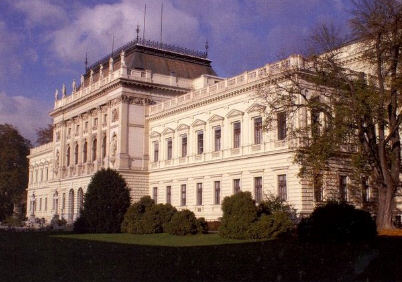# over Cyclic Cubic Number FieldsHistorical Introduction

In the present investigation, we are re-entering uncharted waters.
In 2002, Aïssa Derhem and ourselves analyzed the relations between
the absolute Galois group g = Gal(F31(K*)|Q)
of the Hilbert 3-class field of the absolute 3-genus field K* = (K|Q)*
of cyclic cubic fields K whose conductor f is divisible by two primes (t = 2)
and the norms of primitive ambiguous principal ideals of K.

First we revisited the scenario of Ayadi's Thesis , where both fields
of the doublet sharing the common conductor f have 3-class number h = 9
and 3-class group of type (3,3). In this case, the metabelian 3-group g turned out to be
of maximal class (coclass 1) .

Then, however, we pushed forward to the rare cases, where the members
of the doublet have class numbers divisible by 27 and 3-class group
of type (3,9) or (9,9) or (9,27). In contrast to exact 3-class number 9,
the members may have different 3-class numbers, as we observed in our
primary construction process
using Voronoi's algorithm and the Euler product for class number computation.
Then we proved that, for 27 | h, the Galois groups g are
of second maximal class (coclass 2) ,
and in the particularly hard boiled case f = 42127 even of coclass 3 .
Since this required the computation of the class number of the bicubic field K*,
we gratefully acknowledge that Karim Belabas aided us by means of PARI/GP.

The New Challenge

Now, in the year of our mission Research Frontier 2013, our intention is
to use MAGMA for a break through which would have been impossible in 2002.
Instead of g we are going to determine the relative Galois group
G = Gal(F32(K)|K) of the second Hilbert 3-class field of K
and to show that there are connections between the metabelian 3-groups g and G.

� 1. Possible second 3-class groups, G = G32(K)

It's a lucky coincidence that we've just started an extensive search for
finite p-groups which may occur as higher p-class groups Gpm(K)
of various types of algebraic number fields K, for m ≥ 2.
Thus we are able to give a list of metabelian 3-groups G having G/G' ≅ (3,9)
referring to our latest view of coclass trees for the adopted notation.

G G/G' cl cc TKT Mi TTT G'/G'' dl Aut σ Rank Desc
<33,2> (3,9) 1 2 (0000) <32,1>3,<32,2> (9)3,(3,3) 1 1 2233 1 1/3 2/1
<34,3> (3,9) 2 2 (0000) <33,2>3,<33,5> (3,9)3,(3,3,3) (3) 2 2235 1 3/4 9/5;18/16;4/4
<34,6> (3,9) 2 2 (1111) <33,1>3,<33,2> (27)3,(3,9) (3) 2 2134 0 0/2 0/0
<35,13> (3,9) 3 2 (0004) <34,3>3,<34,15> (3,9)3,(3,3,3,3) (3,3) 2 2237 1 1/4 7/4
<35,14> (3,9) 3 2 (0004) <34,3>3,<34,11> (3,9)3,(3,3,9) (3,3) 2 2237 1 1/4 6/2
<35,15> (3,9) 3 2 (0000) <34,3>3,<34,11> (3,9)3,(3,3,9) (3,3) 2 2237 1 1/4 6/2
<35,16> (3,9) 3 2 (0003) <34,6>3,<34,11> (3,9)3,(3,3,9) (3,3) 2 2136 1 0/3 0/0
<35,17> (3,9) 3 2 (0000) <34,3>2,<34,11>,<34,12> (3,9)2,(3,3,9),(3,3,3) (3,3) 2 2236 1 1/4 7/2
<35,18> (3,9) 3 2 (0040) <34,3>2,<34,11>,<34,13> (3,9)2,(3,3,9),(3,3,3) (3,3) 2 2136 1 0/3 0/0
<35,19> (3,9) 3 2 (0010) <34,6>2,<34,5>,<34,14> (3,9)2,(3,27),(3,3,3) (3,3) 2 2235 1 0/3 0/0
<35,20> (3,9) 3 2 (0010) <34,6>2,<34,5>,<34,14> (3,9)2,(3,27),(3,3,3) (3,3) 2 2235 1 0/3 0/0
<36,9> (3,9) 3 3 (0004) <35,32>3,<35,62> (3,3,9)3,(3,3,3,3) (3,3,3) 2 2239 1 3/5 15/10;61/61;37/37
<36,10> (3,9) 3 3 (0444) <35,32>4 (3,3,9)4 (3,3,3) 2 2238 1 2/4 12/8;13/13
<36,11> (3,9) 3 3 (0440) <35,32>4 (3,3,9)4 (3,3,3) 2 2238 1 2/4 12/8;13/13
<36,12> (3,9) 3 3 (4444) <35,32>3,<35,63> (3,3,9)3,(3,3,3,3) (3,3,3) 2 2139 1 2/4 6/4;9/9
<36,13> (3,9) 3 3 (0113) <35,49>,<35,12>2,<35,35> (3,3,9),(3,27)2,(3,3,9) (3,3,3) 2 2137 1 1/3 5/5
<36,14> (3,9) 3 3 (4233) <35,49>,<35,12>2,<35,36> (3,3,9),(3,27)2,(3,3,9) (3,3,3) 2 2137 1 0/2 0/0
<36,15> (3,9) 3 3 (4323) <35,49>,<35,12>2,<35,36> (3,3,9),(3,27)2,(3,3,9) (3,3,3) 2 2137 1 0/2 0/0
<36,16> (3,9) 3 3 (1114) <35,12>3,<35,64> (3,27)3,(3,3,3,3) (3,3,3) 2 2238 1 1/3 4/4
<36,17> (3,9) 3 3 (1234) <35,12>3,<35,34> (3,27)3,(3,3,9) (3,3,3) 2 2238 1 1/3 3/3
<36,18> (3,9) 3 3 (1320) <35,12>3,<35,34> (3,27)3,(3,3,9) (3,3,3) 2 2238 1 1/3 3/3
<36,19> (3,9) 3 3 (1114) <35,12>3,<35,64> (3,27)3,(3,3,3,3) (3,3,3) 2 2238 1 1/3 4/4
<36,20> (3,9) 3 3 (1324) <35,12>3,<35,34> (3,27)3,(3,3,9) (3,3,3) 2 2238 1 1/3 3/3
<36,21> (3,9) 3 3 (1230) <35,12>3,<35,34> (3,27)3,(3,3,9) (3,3,3) 2 2238 1 1/3 3/3

In the sequel, we shall encounter exactly the same 55 conductors f < 105
of cyclic cubic fields K with 3-class group Cl3(K) of type (3,9)
which we know from our different investigations in 2002.
Only the particularly extravagant conductor f = 42127 is missing,
since both fields of the corresponding doublet have class number divisible by 81.

There are no conductors divisible by three primes (t = 3) such that
one of the associated four cyclic cubic fields is of type (3,9).

The conductors f in the following Sections � 2.1 and � 2.2
completely contain those conductors f = p*q (t = 2) which are given in
Table 3 on p. 41 of the Section "Tables Numériques" in the paper by George Gras .

� 2.1. The 13 conductors f divisible by nine, 9 | f

Here, the members of a doublet have equal 3-class numbers 27
and they also have the same TKT a.1, κ(K) = (0000),
and the same 3-class field tower group G32(K) ≅ <81,3>
which we indicate by exponents denoting iteration.

No. f factors TKT κ(K) G32(K)
8 20421 32*2269 a.12 (0000)2 <81,3>2
15 35163 32*3907 a.12 (0000)2 <81,3>2
24 44397 32*4933 a.12 (0000)2 <81,3>2
25 49743 32*5527 a.12 (0000)2 <81,3>2
30 56223 32*6247 a.12 (0000)2 <81,3>2
32 58329 32*6481 a.12 (0000)2 <81,3>2
36 64971 32*7219 a.12 (0000)2 <81,3>2
40 69183 32*7687 a.12 (0000)2 <81,3>2
42 72099 32*8011 a.12 (0000)2 <81,3>2
46 78093 32*8677 a.12 (0000)2 <81,3>2
47 81009 32*9001 a.12 (0000)2 <81,3>2
49 89109 32*9901 a.12 (0000)2 <81,3>2
52 95913 32*10657 a.12 (0000)2 <81,3>2

� 2.2. The 42 conductors f coprime to three, (f,3) = 1

The members of a doublet do not necessarily have equal 3-class numbers 27.
This forces us to introduce three categories of doublets.

Category I: both members have 3-class group (3,9).
Category II: only a single member has 3-class number 27, the other has at least 81.
Category III: none of the members has 3-class group (3,9).

However, for Category I there arises an additional complication:
In general, the members with 3-class number 27 neither have the same TKT κ(K)
nor the same 3-class field tower group G32(K).
Therefore we use exponents denoting iteration.

No. f factors TKT κ(K) G32(K)
1 4711 7*673 a.12 (0000)2 <81,3>2
2 5383 7*769 b.152 (0004)2 <243,14>2
3 11167 13*859 a.12 (0000)2 <81,3>2
4 12403 79*157 b.152 (0004)2 <243,14>2
5 12439 7*1777 a.12 (0000)2 <81,3>2
6 16177 7*2311 a.12 (0000)2 <81,3>2
7 17593 73*241 a.12 (0000)2 <81,3>2
9 21763 7*3109 b.15,A.20 (0004),(4444) <243,13>,<729,12>
10 25963 7*3709 a.12 (0000)2 <81,3>2
11 27571 79*349 a.12 (0000)2 <81,3>2
12 28177 19*1483 b.152 (0004)2 <243,14>2
13 32311 79*409 b.152 (0004)2 <243,14>2
14 32689 97*337 a.12 (0000)2 <81,3>2
16 36667 37*991 E.12 (1234) <729,17|20>↓
17 37933 7*5419 a.12 (0000)2 <81,3>2
18 38503 139*277 b.15,E.12 (0004),(1234) <243,14>,<729,17|20>
19 40573 13*3121 a.12 (0000)2 <81,3>2
20 40873 7*5839 a.12 (0000)2 <81,3>2
21 41977 13*3229 a.1 (0000) <243,15>
42127 103*409
22 42991 13*3307 a.1 (0000) <243,15>
23 43081 67*643 a.12 (0000)2 <81,3>2
26 49849 79*631 b.15,E.12 (0004),(1234) <243,14>,<729,17|20>
27 51847 139*373 a.12 (0000)2 <81,3>2
28 55657 7*7951 b.15,E.12 (0004),(1234) <243,14>,<729,17|20>
29 55951 7*7993 a.12 (0000)2 <81,3>2
31 57811 13*4447 b.152 (0004)2 <243,13>2
33 59803 79*757 b.152 (0004)2 <243,14>2
34 59911 181*331 b.152 (0004)2 <243,13>2
35 62257 13*4789 a.12 (0000)2 <81,3>2
37 65383 151*433 a.12 (0000)2 <81,3>2
38 66829 7*9547 a.12 (0000)2 <81,3>2
39 68857 37*1861 b.15 (0004) <243,14>↓
41 71611 19*3769 a.12 (0000)2 <81,3>2
43 73873 31*2383 a.12 (0000)2 <81,3>2
44 75859 7*10837 A.202 (4444)2 <729,12>2
45 77281 109*709 a.12 (0000)2 <81,3>2
48 84103 31*2713 b.152 (0004)2 <243,14>2
50 89863 73*1231 a.12 (0000)2 <81,3>2
51 94357 157*601 a.12 (0000)2 <81,3>2
53 96709 97*997 a.12 (0000)2 <81,3>2
54 96817 7*13831 a.12 (0000)2 <81,3>2
55 97249 79*1231 b.15 (0004) <243,14>↓

Successful Conclusions

Our more than 10 years old theory of the Second 3-Genus Group g = g32(K) = Gal(F31(K*)|Q)
is perfectly fitting together with the newly discovered structure of the
Second 3-Class Group G = G32(K) = Gal(F32(K)|K)
of cyclic cubic base fields K whose conductor f is divisible by exactly two primes (t = 2).

First we should point out that the abelianization g/g' ≅ Gal(K*|Q)
is of fixed type (3,3), since the 3-genus field K* of K is bicyclic bicubic,
whereas the abelianization G/G' ≅ Cl3(K) varies in dependence
on the cubic residue characters between the prime divisors of the conductor
and may be of type (3), (3,3), (3,9), (9,9), (9,27), …
having the structure of some nearly homocyclic abelian 3-group.

The possibilities for G provide a refinement of the classification by g
which is given in the following table:

cc(g) g g/g' Parry matrix cc(G) G G/G'
1 <9,2> (3,3) (1010) or (1121) 0 <3,1> (3)
1 <27,3> (3,3) (1111) or (2121) 1 <9,2> (3,3)
1 <81,7> (3,3) (1010) 1 <27,4> (3,3)
2 <243,3> (3,3) (1001) or (1011) 2 <81,3> (3,9)
2 <729,34|37> (3,3) (1010) or (1121) or (2121) 2 <243,14> (3,9)
2 <243,13> (3,9)
3 <729,12> (3,9)
3 <729,17|20> (3,9)
2 <729,40> (3,3) 2 <243,15> (3,9)
2 <729,40>↓ (3,3) 2 <243,14>↓ (3,9)
2 <2187,247>↓ (3,3) 3 <729,17|20>↓ (3,9)
3 <2187,64>↓ (3,3) (9,9) or (9,27)* Web master's e-mail address: contact@algebra.at *